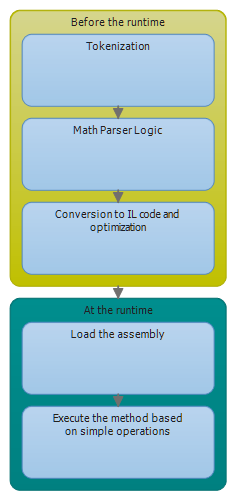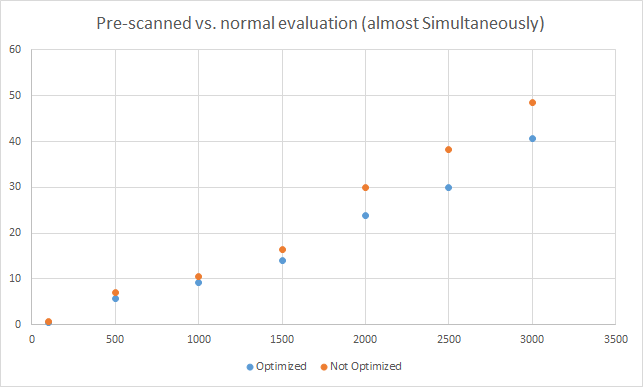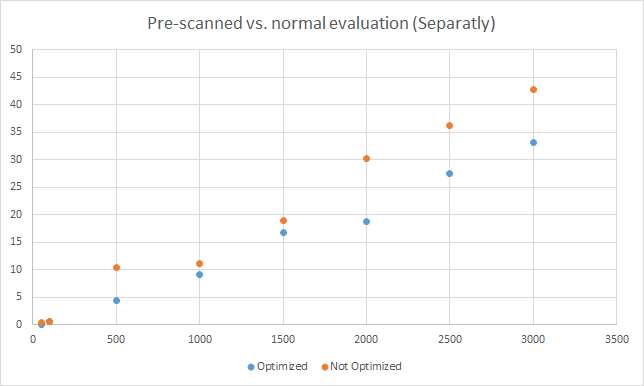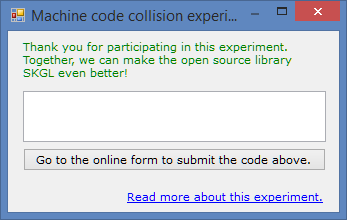# Artem's blog

Mainly .NET (C#, ASP.NET) and my projects

# Release notes for SKGL Extension (2.0.5)

Development of our new Web API is going quite fast. Therefore, it is important to keep SKGL Extension up-to-date so that you can take an advantage of all new features! Today, I want to mention some of the changes that were added to SKGL Extension since 2.0.4.

### Changes

• Get Activated Machines is a new method in the Web API (see here) that allows you to get all the activated devices (machine code, IP and Time) for any given key. Note, you need to explicitly enable it as well as use your privateKey for each request. This method has the same authentication level as key generation. An example is shown below:

As you can see, in contrast to the classical operations such as validation and activation, pid, uid and hsum are passed in as a JSON array. I hope that all methods will work that way as soon as possible, because it is very easy to simply copy and paste from here and click on “Get C# friendly version”.
• Load and Save Key Information to File will from now on take care of all error handling. This means that you need not to surround these method calls with a try-catch-finally. It’s great because you don’t need to worry if the stream is open during an exception.

# Release notes for SKGL Extension (2.0.4)

I am happy to tell all users of SKGL Extension that there is a new version available (since the update in late January). In this post, I would like to mention some changes made to the API, a short usage tip, and then let you know how it is going to change in future.

### Changes

• Ability to sign pid, uid, activation dateBased on user feedback, the Web API now allows pid, uid and hsum to be added into the signature. This is particularly important for users that protect more than one product. Before, it was possible to use the same KeyInformation file (serialized by SaveKeyInformationToFile) to unlock other products (that used the same Public Key, i.e. by the same software vendor). For some, it worked out by specifying this information in the Notes field. This change makes it more simple. The activation date is intended for offline key validation; it’s a way to keep track the date of the last activation in order to force the user to activate the software periodically.
• Deactivation: There is a new method that allows you to deactivate a license key. (method info, web api)
• Optional Field: Finally you can access the optional field using .NET.  It’s a new type of method that takes in a ProductVariables object instead of separate strings for pid, uid, hsum, etc. Read more below Future ideas  (more info).
• Machine code fix: The getEightDigitsLongHash was modified to fix a bug that generated nine digits instead of eight. Read more here.
• Proxy: Get Parameters method allows you to specify the proxy settings.
• Load Product Variables: A new method, LoadProductVariablesFromString allows you to load a serialized version of the ProductVariables object. Read more below Future ideas.

### Usage tip

Some users have encountered problems when validating/activating keys because of a wrong code snippet (NullReferenceException). Basically, you should not check the .IsValid field as way to deduce whether the key is valid or invalid. Instead, check if the KeyInformation object is null.

### Future ideas

As new functionality is added to the Web API,  more parameters have to be added to each method. In the long run, this is bad because it can be quite repetitive/confusing to add all the parameters all over again. My plan on how this can be resolved is by adding specialized classes, such as ProductVariables to facilitate reuse of existing variables in other methods. The goal is to make it as easy as possible to use SKGL Extension.

# The Power of Finite Differences in Real World Problems

## Introduction

Most of the people are certainly familiar with Calculus already from high school and the beginning of university. It is usually associated with concepts like infinitely smallbehaviour at infinity, finding the area under the graph, and finding the rate of change. It seems to be so much about infinity (which is difficult to imagine) but not that much about finite values. So why not look at finite differences instead? In this article, we are going to see how powerful the concept difference is, and how we can apply it to real world problems. First, we are going to look at how the difference can be found in a sequence. Secondly, the way we can find the next term and later on how we can get a formula to generate the nth term. Finally, we are going to use the idea of finding the nth term to find an expression of the sum.

## The difference operation

It is quite intuitive, once we see a sequence, to try to find the difference between adjacent terms. If we are not satisfied, we look at the difference of the differences, and so on. Our aim now is to construct a method that will behave like we normally would in this situation. First, however, let’s look at what happens when we take the difference of each term.

 $$a_0$$ $$a_1-a_0$$ $$a_1$$ $$a_2-2a_1+a_0$$ $$a_2-a_1$$ $$a_3-3a_2+3a_1-a_0$$ $$a_2$$ $$a_3-2a_2+a_1$$ $$a_3-a_2$$ $$a_3$$

We can take the difference as many times as we like, but given that a sequence was constructed with only addition, subtraction, multiplication and division i.e. it was a polynomial, there is going to be a point when we can stop taking the difference. The important thing now to realize from this figure is that the difference expression contains the coefficients from the Pascal’s triangle. This is good if we want to take the difference many number of times. The code below utilizes this observation.

## Finding the next term

Before we proceed in finding the next term and ultimately the polynomial describing the nth term, let’s focus our attention on a real world example. Say we have the sequence $$\{n^2\}$$ i.e. $$1, 4, 9, 16 \dots$$. We assume that we don’t know the nth term, i.e. we only know some values of the sequence. The common procedure in this case is to look at the differences, similar to the previous section. For example,

 $$1$$ $$3$$ $$4$$ $$2$$ $$5$$ $$0$$ $$9$$ $$2$$ $$7$$ $$16$$

To be sure that we’ve found a pattern, we should end up at zero (assuming a polynomial generated the sequence). The interesting thing about this figure is that we know that each difference in the table can be expressed in terms of the difference expressions in the previous section. So, $$3=(4)-(1)$$ and $$2 = (9)-2\times (4)+(1)$$. This idea hints us to a possible way of finding the next term in the sequence. Since we know that the second difference $$2$$ is constant, we can find an expression for the next term by some simple arithmetic. That is, $$2=a_n -2\times a_{n-1} + a_{n-2}$$.  Thus we can find the nth term given the two terms before it. This is done by the following code:

## Finding the expression for the nth term

This is a bit more tricky to find in contrast to what we’ve done so far. The nth term is very dependent on the number of times the difference operation has to be taken before we end up at zero. In the previous example, we had to take it three times to get to zero. The polynomial we got had the second degree. It turns out that if we had to take the difference $$n$$ times to get to zero, the polynomial is of the degree $$n-1$$. This is quite useful, because if we know how the polynomial looks like, the only thing we need to find are the coefficients (more in-depth tutorial).

In the sequence $$1,4,9,16\dots$$, we had to take the difference three times, so the polynomial has the second degree, i.e. $$ax^2+bx+c$$. We have three unknowns, so we need three data points to solve the system of equations. That is,

$$\left[ {\begin{array}{*{20}{c}} 1 & 1 & 1 & 1 \\ 4 & 2 & 1 & 4 \\ 9 & 3 & 1 & 9 \end{array}} \right]$$

When we reduce this matrix to echelon form, we get that the coefficient for $$n^2$$ is $$1$$, and zero for the remaining terms. The code below does this task.

The coefficients we get correspond to a term in the polynomial. For example, if we get $$3, 2, 1$$, the polynomial is $$3x^2+2x+1$$.

## Finding the closed form for the sum

When we first think about how we can find the closed form for a sum, we might conclude that it is difficult. In Concrete Mathematics – A Foundation for Computer Science, an entire chapter (chp. 2) and a great part of other chapters is dedicated to sums, and the way we can find the anti-difference. At least, that was my thought when I got this idea. But later, an interesting thought came up to my mind, and that is to treat the sum as a sequence and the sequence as the difference of the sums’ terms. Let’s clarify this. If we have the sequence $$1,2,3,4,\dots$$, we can construct the partial sums, i.e. $$1, 1+2, 1+3+4, 1+2+3+4$$, thus $$1, 3, 6, 10$$. But the partial sums form a new sequence which we can analyse in a similar way. This means that can we can reuse quite a lot of code. Now, since in the original sequence $$1,2,3,4,\dots$$, we have to take the difference twice to get zero, in the new sequence $$1, 3, 6, 10$$, we have to take the difference three times. The code for doing this is shorter, in contrast to the previous ones.

## Limitations

One of the limitations of this code/algorithm is that we can only use sequences that have been generated by a polynomial. Exponents are not allowed, although $$n^2= n\times n$$, so that works. The reason is quite simple. Formally, we define difference as an operator on a function (similar to derivatives) as

$$\Delta f(x) = f(x+1) – f(x)$$. Let’s say we have $$f(x)=2^x$$. If we take $$\Delta(2^x)=2^{x+1}-2^x=2^x(2-1)=2^x$$. Not good, no matter how many times we take the difference, we end up with the same thing we had in the beginning. This is why this particular algorithm does not work in this case. It is possible to add an extra step that checks against known sequences each time a difference is taken. This, I will do a bit later! 🙂

## Source code

You can find all additional methods in the FiniteCalculus class in Mathos.Calculus (Mathos Core Library). Also, you can see this code in action here!

# Mathos Parser is now an expression compiler

The requirement of performing several thousands of calculations (for example, during integration) led to the optimization with pre-scanned expressions. But the aim to make Mathos Parser even faster still remained. Now, I would like to introduce you to a new project of Mathos Project – Mathos Expression Compiler, based on the math tokenizer and parser logic of Mathos Parser.

This API allows you to parse an expression and “store” it as CIL code. Then, using ILAsm, you can generate a new assembly that will contain your expression entirely parsed. The only operation that occurs at runtime is the insertion of values into the variables that you declared and the operation on that variable value. To make it even faster, most of the calculations are already done before the runtime, for example, x+3+5 would be stored as x+8.

In this scenario, the time consideration can be important. Even if it is faster to execute CIL instructions, the compilation process can take some time. This has not been tested yet. The process is illustrated below:This is a very simplified way of looking at it but the point is to always test Mathos Parser together with Mathos Expression Compiler and make an estimate of how much time would be saved in different scenarios. It all depends on the context. But note, that

A performance hit is incurred only the first time the method is called. All subsequent calls to the method execute at the full speed of the native code because verification and compilation to native code don’t need to be performed again. //p. 12. Jeffrey Richter. CLR via C# 3rd Edition

So, if you plan to execute the function trillion times, it might be a good a idea to keep in mind the Expression Compiler as a better alternative, because at that point, the compilation time will be negligible.

Now, back to the features. It was mentioned in the beginning that it is based on the Mathos Parser. Note, in terms of features, Mathos Expression Compiler has at this point only a subset of those of Mathos Parser. At this point, you cannot have functions as sincos, and your own custom functions, but this will be changed in the nearest future.

There are also some changes to the way operators work. To declare an operator properly, you should use the variables OperatorListOperatorAction, and OperatorActionIL. Below, a simple example of addition operator:

Comparison operators are a bit more tricky. An example of greater than operator is shown below:

For those who understand IL, the @ sign might seem a bit strange. It is simply a way to tell the stack count to the if statement (in the compilation process).

When you actually generate an expression, this is how your IL code for that expression might look:

Will return (only IL for the expression):

When you have the IL code, you should name the output executable(or dll) as MathosILParser, otherwise, you might get an error. In future, an option will be added to customize the name of the output file, but at this point, please use RegEx (or replace option) to change the MathosILParser.exe to something else (in the IL code).

Your function can later by called as shown below. Note, I am not using reflection at all.

I hope this short guide was helpful, even if it might be a bit messy. If you have performed any testing related to speed or if you want to show some examples, please feel free to comment or contact me.

# The advantages of pre-scanned expressions

RubyInt project, or Iron Ruby Based Mathos Core Library Interpreter was one of the projects to be tested with the new parser feature, that is, pre-scanned expressions.

In the Integral approximation module, it is quite good to use pre-scanned expressions, since the expression stays the same through the entire process. The execution time improved in this case.

The integral approximation website in Mathos Laboratory (see it here) has also shown good results thanks to this optimization. In Mathos Laboratory in particular, it is important that methods are quick and use as small amount of resources as possible, because it is hosted in the Cloud.

I have performed some quick tests using an i3 processor (the methods are in changeset 37964), although I would not recommend them to draw specific conclusions (there is a random error involved so more repetitions of each test are needed). Also, the difficulty level of an expression can affect the tokenization time. The graphs are located below:One conclusion that can be drawn from this is that the optimization only gets useful when the same expression is evaluated more than 100-500 times.

# Faster evaluation using a pre-scanned expression

[This article was updated to included changes in v. 1.0.7.2. Originally written for v. 1.0.7.1]

Today, I published a newer version of Mathos Parser on both CodePlex and NuGet. The release notes:

from v.1.0.7.2:

• Force user to use ReadOnlyCollection when using a token list as a parameter.

from v. 1.0.7.1

• Changed the structure a bit. Now, the Scanner returns tokens instead of continuing with MathParserLogic. This is good if the same kind of expression is to be used many times.

But what does this really mean? Well, previous versions of the parser worked more locally, that is, once one function was executed, it triggered another function, and so on. Now, however, the Scanner (private method) returns a list of tokens instead of launching MathParserLogic directly. If you simply want to get tokens, you would have to execute the GetTokens function, as shown below:

You can then send the tokens list into either Parse or ProgrammaticallyParse and it will parse it in the same way as if you would enter a string expression, that is:

Both statements above will return 32, but there is one main difference; the execution time will be so much smaller for the second statement, where a “pre-compiled” list is being used. If it would take 100 milliseconds (ms) to find the value of the first statement, it would take approximately 9-10 ms for the second one. The ratio is 10:1.

This is great if you want to perform an operation where the same function is involved several thousands times, for example when approximating an integral.

I am currently thinking about converting math expressions into IL code, but this will happen sometime in future!

# Creating a programming language with Mathos Parser (2)

In this article, I would like to find out whether it is possible to construct a programming language with Mathos Parser. In general, the question is if a simple expression parser has the necessary things to easily convert it into a language.

The answer is yes, but the language will be very limited if additional features are not added. The reason for this is because what most languages have in common is some sort of  user/machine interaction, while an expression parser only allows you to parse mathematical things and is not really designed to interpret how to write to a file or allocate memory.

For example, imagine you want to create something similar to if statements  and you decide to treat an if as a function. You would use following code:

So, if whatever we pass into the first parameter is true, the value in the second parameter will be returned. This is quite cool, but it does not allow us to return a series of statements. Instead, we could design a new kind of if statement that would, depending on the result from this if function, execute the right method. Here is how I solved it:

As you can see, the if statement is still there, but there is now a feature that will interpret the result and make something happen. You can also use \n to separate between different statements and also name a collection of statements, as shown below:

Note that both examples use a function get(). It can also be implemented as a function:

You might have noticed the use of statements (see notTrue statement). Their role is to simulate a method. Everything you enter into a statement will not be executed until you call it, so you can insert undefined variables into a statement when you define it and later assign them with a value. I have also added commands like write, title, pause, clear and end. These are extensions to the language and are interpreted in the method below:

Some programmers will certainly argue that the code above is not that optimized and is quite primitive. Well, it serves its purpose and makes Mathos Parser look more like a language.

In the next article I want to implement a while feature into this awesome programming language.

Conclusion: Just because something can parse expressions does not mean it can parse a series of statement. In order to fix this we can either choose to add some features externally, or, go into the parser itself and make it more adjusted to this particular task. This language does not alter Mathos Parser in any way.

# Factorial notation in Mathos Parser (1)

In this article series, I would like to look at how Mathos Parser (a very simple expression parser) can be used to perform things that were not originally intended. Today, I am going to show what has to be done to make Mathos Parser able to interpret factorial notation, such as “4!

Already in the parser, we can solve this in at least two different ways:

• creating a localFunction fact() that will take one parameter and return the factorial of that number
• using FactorialPower(falling power) instead, that is 4!4 would be 24 and 4!1=4.

Both methods do not require you to change anything in the input string, but they might not be as close to the mathematical notation as we want it to be. Therefore, as a part of this article, I am going to illustrate the changes in the input string that have to be made, to make it understand 4!.

1. Include Reg Ex name space as following:
2. Add these two methods somewhere in the class:
3. Define the Mathos Parser and add a definition of the factorial operator including the way it should be executed. Note, at this stage, for this to work, the factorial number has to be in the form of 4!0 or 4!1 ((both result in 24).
4. Before the input string can be parsed, perform the following action (assuming you have defined a variable input with a value):
5. Parser the string and store the result:

This is how we can make Mathos Parser able to understand mathematical notation of factorial. In the next article of this series, we are going to look at how Mathos Parser can be used to construct a new programming language.

# A possible change for machine code

In the recent issue, it was reported that machine codes are repeated approximately once per 50 different machines in SKGL 2.0.5.3. The reasons under investigation are:

• small hash value allows even more collisions.
• the machine information that is being hashed does not collect all hardware that can be changed.

The first reason is currently in focus, and I have tried to develop a piece of code that now increases the length of the machine code. It would be great if those of you who are able to test this on several machines could do so, and comment(either directly below this thread or here) how frequent the collisions are on different machines! Thank you in advance! 🙂

EDIT 2: This can be done by anyone using Windows: